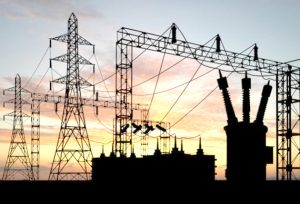# Electrical Power System TrainingThis course is an introduction to the field of electric power systems. Material covered in the subject includes: Fundamentals of energy conversion system. Modeling of main power network components such as power transmission lines, transformers and synchronous machines. Basic description of their behavior using appropriate representations. Simplification of problems using transformation techniques. Fundamentals of network analysis, power system representation, power load flow. Review of symmetrical components and short circuit analysis.

#### OBJECTIVE

* Describe of the common methods of converting energy to electric power.
* Descrive the classical power system structure.
* Perform steady-state analysis for a balanced three-phase power system.
* Represent elements of a power system including generators, transmission lines, and transformers.
* Understand the functioning of a synchronous machine and represent it with simple models.
* Construct a composite system by the interconnection of the elements of a power system.
* Analyze multi-node power systems using an admittance matrix or impedance matrix representation of the power system.
* Factor the admittance matrix to obtain a solution of the network voltages.
* Generate the elements of the impedance matrix from the elements of the admittance matrix without a matrix inversion.
* Understand the formulation of the power flow problem, and have the ability to cast any given system in this framework.
* Solve power flow problems by application of the Newton method.
* Design a transmission addition to a power system using tools and methods from the course.
* Formulate a mathematical model for analyzing faults on power systems.

#### CONTENT

1. Introduction to Electrical Energy System

* Sources of Energy
* Electromechanical Energy Conversion
* Environmental Impact of Electricity Generation and Transmission
* The Generation Subsystem
* The Transmission Subsystem
* The Distribution Subsystem
* Power Industry Structure

2. Introduction to Polyphase Networks Analysis

* Balanced Three-Phase Circuits :

+ Delta Connection
+ Wye Connection
+ Power Calculations in Balanced Three-Phase Circuits
+ Per-Phase Analysis

3. Transformers and Per-Unit Systems

* The Ideal Transformer
* Three-Phase Transformer
* An Actual Transformer
* Introduction to Per-Unit Systems

4. Electric Power Generation and Transmission Systems

* Electric Power Generation
* Electric Power Transmission

+ Transmission Line Components
+ Complex Power Transmission

* Power Flow
* The Power Flow Problem
* Newton-Raphson Iteration
* Application to Power Flow Equations

#### METHOD

Presentation

Discussion

Practice

Case Study

Evaluation

Electrical Power System Training
Tanyakan pada kami ?We are here to help you! Do not hesitate to ask us anything. Click below to start chat.Marketing

### Aini

OnlineMarketing

### Maulana

OnlineAini

Hai, ada yang dapat kami bantu ? 00.00Maulana

Hai, tanyakan pada kami ? 00.00